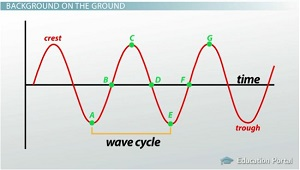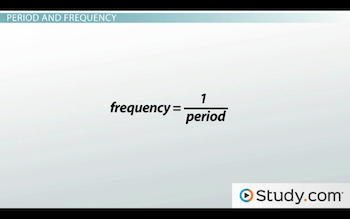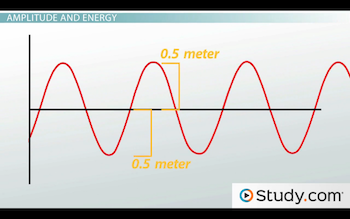# Wave Parameters: Wavelength, Amplitude, Period, Frequency & Speed

An error occurred trying to load this video.

Try refreshing the page, or contact customer support.

Coming up next: Transverse & Longitudinal Waves: Definition & Examples

### You're on a roll. Keep up the good work!

Replay
Your next lesson will play in 10 seconds
• 1:24 Period and Frequency
• 3:16 Amplitude and Energy
• 4:10 Speed and Wavelength
• 6:02 Lesson Summary

Want to watch this again later?

Timeline
Autoplay
Autoplay
Speed

#### Recommended Lessons and Courses for You

Lesson Transcript
Instructor: April Koch

April teaches high school science and holds a master's degree in education.

How do we measure and describe waves? How do waves differ based upon their shapes? This lesson will guide you through the five wave parameters - period, frequency, amplitude, wavelength, and speed - that we use to characterize waves.

## Wave Parameters

So, we've learned a little bit about waves, right? We've learned that waves originate from vibrations, which are oscillating motions over a fixed position. A vibration can cause a disturbance to travel through a medium, transporting energy without transporting matter. This is what a wave is. But, how do we properly talk about waves? How do we compare them to one another? Can we measure the size and speed of a wave? How do we know how much energy it carries?

To find out, we'll need to look at the major wave parameters: the ways in which we measure waves. We'll learn how to characterize a wave by its period, frequency, amplitude, speed, and wavelength. Once we get to know the right way to use these parameters, we'll be able to learn more about how the different waves work.Let's start off by remembering what a wave looks like. We've seen the picture above before. It's a wave drawn over a set of X and Y axes. We plotted the wave as a function of time and said that the portion of a wave between two crests or troughs is called a wave cycle. From this image, we can see that waves travel by crests and troughs in a periodic fashion. That is, a full wave cycle always takes the same amount of time. In this case, that amount of time is exactly two seconds. Two seconds is called the period of the wave.

## Period and Frequency

The period is the time it takes a wave to complete one cycle. We measure the period in seconds, and we symbolize it with the capital letter T. You can think of the period as the time it takes for one particle in the medium to move back and forth. If this were a water wave, all the particles in the water would be moving up and down as the wave travels through. The time it takes for one water molecule to move up, move back down, and then return to its original position, is called the period.

Knowing the period of a wave is fine, but we often need to talk about waves in terms of how often the wave cycles are coming. In other words, we want to know the frequency of a wave. A wave's frequency is the number of cycles that are completed in a certain amount of time. The symbol for frequency is the lowercase f, and we measure it in cycles per second, which is the same as the unit hertz. A wave with a frequency of 20 Hz completes 20 wave cycles every second.

Be careful that you don't confuse frequency with period. This is a common mistake. Frequency and period are actually opposites. While period is measured in seconds per cycle, frequency is measured in cycles per second. Consider our wave with a period of 2 seconds. Since the wave completes one cycle every two seconds, then its frequency is one half or 0.5 Hz. So, you see - period and frequency are reciprocals of each other. We can represent their relationship with a simple equation:The greater the period is for a wave, the less wave cycles can fit within a second, and so the lower the frequency gets. Likewise, a wave with a larger frequency would have to fit more wave cycles into every second, meaning the period of each cycle would have to be smaller. No matter what kind of wave you're looking at, the period and frequency will always be inversely proportional to each other.

## Amplitude and Energy

So, now we know how to measure a wave based on cycles and time, but what about the height of a wave? Can we measure how high a wave's crest reaches or how low the trough dips down? We already mentioned the amplitude in our previous lesson. It's the distance between the midline of a wave and its crest or trough. Amplitude measures how much energy is being transported by the wave. The larger the amplitude, the more energy a wave has.

The symbol for amplitude is a capital letter A. Be careful not to make the mistake of thinking amplitude is the distance from crest to trough. It's only the distance from the resting point. Let's take the example of a giant water wave.We can see that the crests reach half a meter above the resting point, and the troughs reach half a meter below the resting point. It doesn't matter whether we look at the crest or the trough. The amplitude for this wave is 0.5 meters.

To unlock this lesson you must be a Study.com Member.

### Register to view this lesson

Are you a student or a teacher?

### Unlock Your Education

#### See for yourself why 30 million people use Study.com

##### Become a Study.com member and start learning now.
Back
What teachers are saying about Study.com

### Earning College Credit

Did you know… We have over 160 college courses that prepare you to earn credit by exam that is accepted by over 1,500 colleges and universities. You can test out of the first two years of college and save thousands off your degree. Anyone can earn credit-by-exam regardless of age or education level.